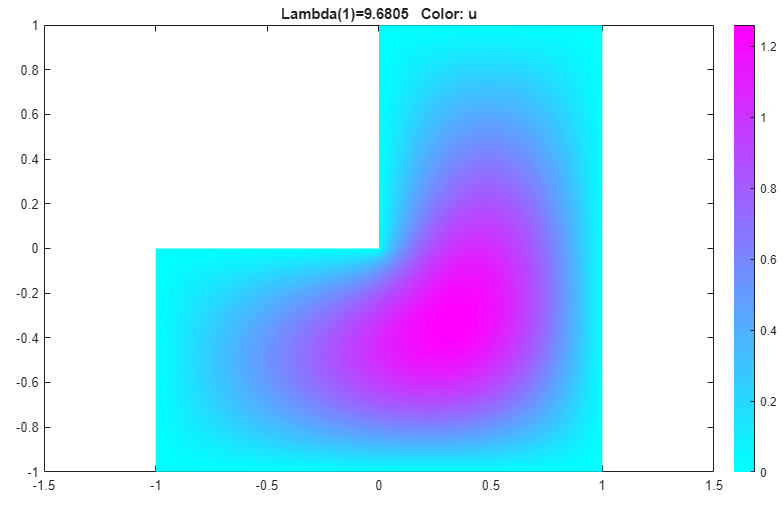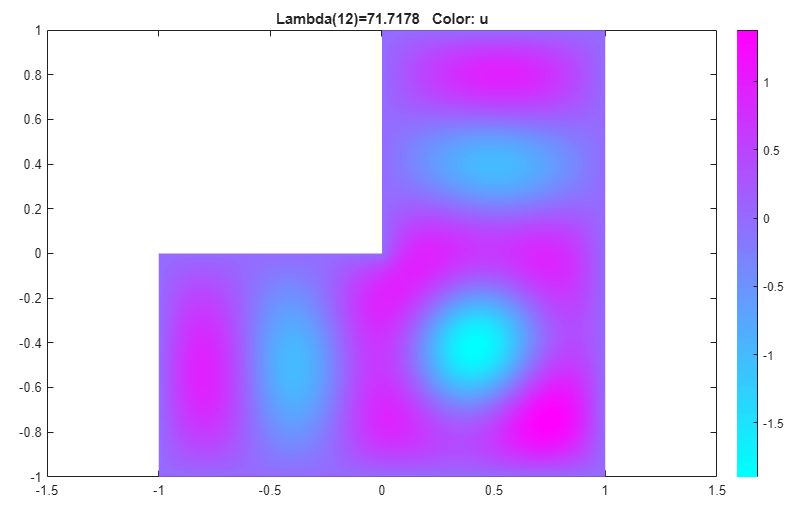## Eigenvalues and Eigenmodes of L-Shaped Membrane: PDE Modeler App

This example shows how to compute all eigenmodes with eigenvalues smaller than 100 for the eigenmode PDE problem

–Δu = λu

on the geometry of the L-shaped membrane. The boundary condition is the Dirichlet condition u = 0. This example uses the PDE Modeler app. For a programmatic workflow, see Eigenvalues and Eigenmodes of L-Shaped Membrane.

To solve this problem in the PDE Modeler app, follow these steps:

1. Draw a polygon with the corners (0,0), (–1,0), (–1,–1), (1,–1), (1,1), and (0,1) by using the `pdepoly` function.

`pdepoly([0,-1,-1,1,1,0],[0,0,-1,-1,1,1])`
2. Check that the application mode is set to Generic Scalar.

3. Use the default Dirichlet boundary condition u = 0 for all boundaries. To verify it, switch to boundary mode by selecting Boundary > Boundary Mode. Use Edit > Select all to select all boundaries. Select Boundary > Specify Boundary Conditions and verify that the boundary condition is the Dirichlet condition with `h = 1`, `r = 0`.

4. Specify the coefficients by selecting PDE > PDE Specification or clicking the PDE button on the toolbar. This is an eigenvalue problem, so select the Eigenmodes type of PDE. The general eigenvalue PDE is described by $-\nabla \cdot \left(c\nabla u\right)+au=\lambda du$. Thus, for this problem, use the default coefficients ```c = 1```, `a = 0`, and `d = 1`.

5. Specify the maximum edge size for the mesh by selecting Mesh > Parameters. Set the maximum edge size value to 0.05.

6. Initialize the mesh by selecting Mesh > Initialize Mesh.

7. Specify the eigenvalue range by selecting Solve > Parameters. In the resulting dialog box, use the default eigenvalue range `[0 100]`.

8. Solve the PDE by selecting Solve > Solve PDE or clicking the = button on the toolbar. By default, the app plots the first eigenfunction.9. Plot other eigenfunctions by selecting Plot > Parameters and then selecting the corresponding eigenvalue from the drop-down list at the bottom of the dialog box. For example, plot the fifth eigenfunction in the specified range.## SupportGet trial now